# Linear algebra software packages

software for linear algebra

Like Euclidean geometry, linear algebra is one of the oldest and most fundamental subjects in mathematics. Its methods are among the most widely used in applications. There is hardly a subfield of applied mathematics where linear algebra is not used, though nowadays (1998) its use may be hidden in software. Remarkably, linear algebra is also a very active field of contemporary research, both for small scale and large scale problems.

Software packages for linear algebra are symbolic, numeric or a combination of both. Symbolic methods perform exact manipulations on numbers or algebraic symbols, while numeric methods deal with floating point representations of numbers on which operations are performed with a limited relative accuracy. It is perfectly reasonable to compute symbolically the Jordan form of a given matrix if the matrix is given exactly and is small or has special structure. On the other hand, the numerical computation of the Jordan form of a numerically given matrix is an ill-posed problem in the sense that it may be infinitely sensitive to perturbations of the matrix (cf. also Ill-posed problems). The "correct" approach is then to compute the distance from the matrix to the set of matrices with a prescribed Jordan type; this is typically a well-posed problem.

Conversely, a dense linear system ofequations in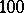unknowns can be solved numerically in a fraction of a second by modern computers, while it is probably unfeasible to do this by symbolic methods.

For recent (1998) surveys of algorithms for numerical linear algebra problems, see [a2], [a3], [a5].

Fundamental problems in numerical linear algebra are:

Solution of linear systems of equations. Given a matrix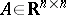and a vector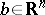, compute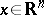such that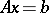.

Solution of least squares and minimum norm problems. Given a matrixand a vector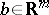, computesuch that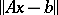is minimal, wheredenotes the Euclidean norm. If the vectoris not uniquely determined by this requirement, then compute among all solutions the one with minimal norm (this one always exists and is unique).

Computation of eigenvalues and eigenvectors. Given a matrix, compute scalarsorand vectors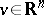orsuch that.

Linear algebra also deals with several subproblems and related problems such as:

The computation of matrix decompositions, such as LU-, QR- and Cholesky decompositions (cf. also Matrix factorization).

Providing estimates for the condition number of a matrix, i.e., of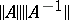where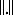denotes an appropriate matrix norm.

The computation of the rank of a matrix, i.e. the number of linearly independent rows and columns.

Generalized eigenvalue problems, i.e. computing,such thatfor given matrices,.

Singular value decomposition, i.e. decomposing a given matrix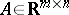as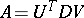where,are orthogonal matrices andis a diagonal matrix with the dimensions ofand with only non-negative numbers on the diagonal; these numbers are unique up to a permutation and are called the singular values of.

There are presently (1998) many software packages for linear algebra problems. Some are interactive, others are not. Symbolic packages are usually interactive and allow also the numerical treatment of small scale problems. Numeric packages are usually non-interactive and consist of collections of subroutines in a programming language, usually FORTRAN, sometimes C or C++. Their main field of application is in algorithms with high numerical robustness and large scale computations.

The widely available general mathematical software packages DERIVE, GAUSS, MAPLE, MATHEMATICA, MATLAB, REDUCE (cf. also Computer algebra package) include facilities to handle linear algebra problems. They provide

Commands to perform low-level linear algebra manipulations such as adding a multiple of a vector to another vector, computing an inner product, etc.

Routines to generate matrices with pseudo-random entries and special types of matrices, such as the Bezout or Sylvester matrix, Jacobian, Wronskian and Hessian matrices.

Routines to compute normal forms, such as the Jordan and Smith normal forms.

Routines to compute determinants and matrix exponentials.

Symbolic and numeric routines to solve the fundamental and related problems for dense matrices and some sparse matrices with special structure, in particular band matrices. The routines are based on direct methods or (for eigenvalue problems) very robust and well-understood iterative methods such as the QR-algorithm.

The leading package of non-interactive, numerical routines for linear algebra computations is LAPACK [a1], which is freely available from the electronic numerical analysis library netlib, [a8]. LAPACK routines are portable codes written so that as much as possible of the computation is performed by calls to the Basic Linear Algebra Subprograms (BLAS). The BLAS are also available from netlib. They perform the low-level linear algebra routines; highly efficient implementations are available for current machines and regularly updated. LAPACK is written in FORTRAN 77 but versions in FORTRAN 90, C and C++ are also available from netlib. The routines in LAPACK are available in specific forms for general dense, band and symmetric matrices and for various variable types (real, double precision real, complex, double precision complex).

LAPACK is also distributed as part of the NAG library.

LAPACK does not contain routines for sparse matrix computations. However, there are several other packages that can either solve linear systems with sparse matrices or compute eigenvalues of sparse matrices or both. Linear systems are either solved by direct sparse methods or by iterative methods. A survey of freely available software is at present maintained at the [a6].

One of these packages is ARPACK (ARnoldi PACKage), a reliable numerical package for the computation of a few eigenvalues of a large sparse matrix. Note that realistic applications of such matrices, i.e. in stability and bifurcation theory, typically do not require all eigenvalues but only a few eigenvalues that lie in a specific region of the complex plane. For the underlying numerical analysis ideas, see [a4]. ARPACK consists of a collection of FORTRAN 77 routines. Important features are:

The user decides how many eigenvalues are wanted and has a choice of possibilities to decide which ones, e.g. those with largest absolute value, largest real part, close to a given number, etc.

The package is independent of the storage structure of the matrices. To perform matrix-vector products or (in some options) solutions with linear systems, control is returned to the driving program (this is called a reverse communication interface).

There are separate routines for symmetric and non-symmetric matrices.

Both standard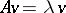and generalized eigenvalue problemscan be solved.

Singular values can also be computed.

ARPACK is a non-commercial product developed by R. Lehoucq, D.C. Sorensen, C. Yang and K. Maschhoff [a7].